## Exercise 2

Knowing that tan α = 2, and that 180º < α < 270°, calculate the remaining trigonometric ratios of angle α.

## Exercise 3

Knowing that sec α = 2 and 0 < α <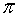/2, calculate the remaining trigonometric ratios of angle α.

## Exercise 4

Knowing that csc α = 3, calculate the remaining trigonometric ratios of angle α.

## Exercise 5

Prove the identities:

12345## Exercise 6

Simplify the fractions:

123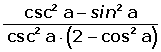## Exercise 7

Prove the identities:

## Exercise 1## Exercise 2## Exercise 8

Simplify the fractions:

## Exercise 1## Exercise 2## Exercise 3## Exercise 9

Calculate the trigonometric ratios of 15 (from the 45º and 30º).

## Exercise 10

Develop: cos(x+y+z).

## Exercise 11

Calculate sin 3x, depending on sin x.

## Exercise 12

Calculate sin x, cos x and tan x, in terms of tan x/2.

## Solution of exercise 1

Knowing that cos α = ¼ , and that 270º <α <360°, calculate the remaining trigonometric ratios of angle α.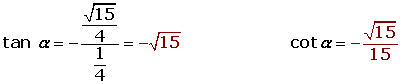## Solution of exercise 2

Knowing that tan α = 2, and that 180º < α <270°, calculate the remaining trigonometric ratios of angle α.## Solution of exercise 3

Knowing that sec α = 2 and 0< α </2, calculate the remaining trigonometric ratios of angle α.## Solution of exercise 4

Knowing that csc α = 3, calculate the remaining trigonometric ratios of angle α.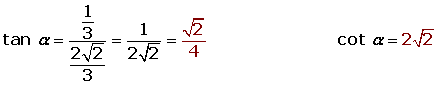## Solution of exercise 5

Prove the identities:

123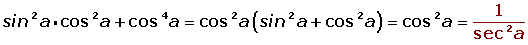45## Solution of exercise 6

Simplify the fractions:

123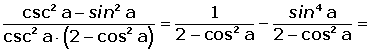## Solution of exercise 7

Prove the identities:

1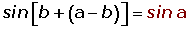2## Solution of exercise 8

Simplify the fractions:

1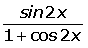23## Solution of exercise 9

Calculate the trigonometric ratios of 15º (from the 45º and 30º).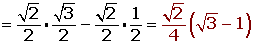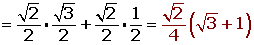## Solution of exercise 10

Develop: cos(x+y+z).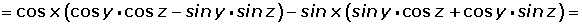## Solution of exercise 11

Calculate sin 3x, depending on sin x.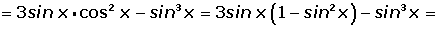## Solution of exercise 12

Calculate sin x, cos x and tan x, in terms of tan x/2.